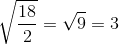# SAT II Chemistry : Gases

## Example Questions

### Example Question #1 : Gas Laws

Suppose that an ideal gas atand 2.0 atm has a volume of 11.2 cubic meters. Which of the following expressions represents the volume of the gas, in cubic meters, if the temperature were increased byand the pressure decreased to 1.5 atm?Explanation:

First of all, the unit degrees Celsius should be converted to Kelvins by adding 273. This means that the temperature increases from 273 K to 323 K.

Next, the combined gas law can be used.Plugging in the known values givesand solving for the volume givesRearranging shows this is clearly equivalent to the correct answer,.

### Example Question #1 : Solutions And States Of Matter

How much faster is the rate of effusion of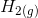than the rate of effusion of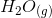?By Graham's Law,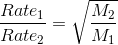. The molar mass of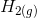is 2 g/mol and the molar mass ofis 18 g/mol. Thus,